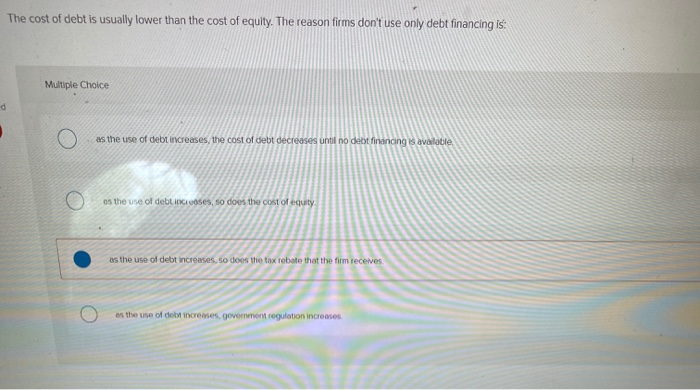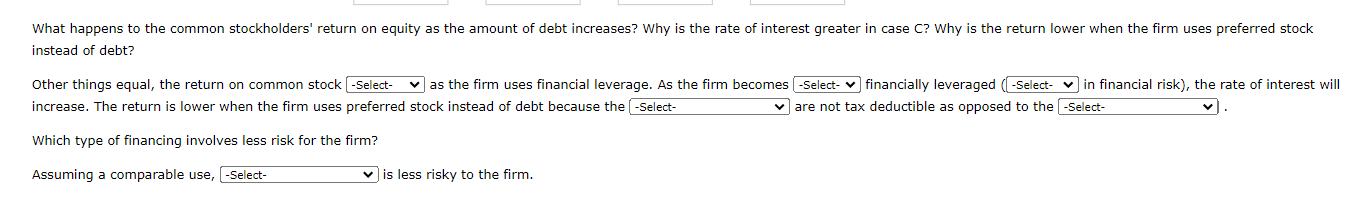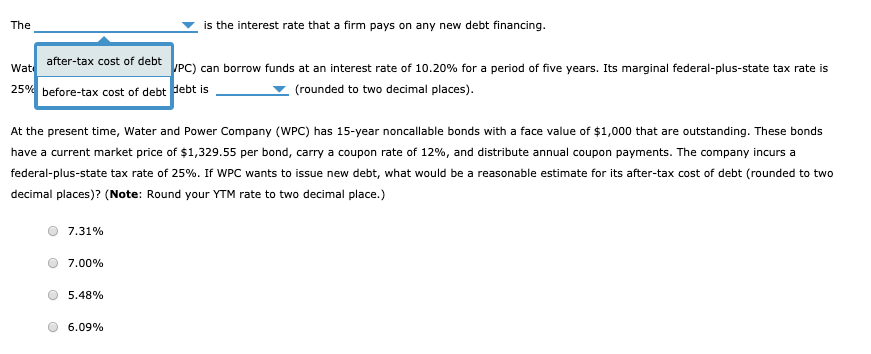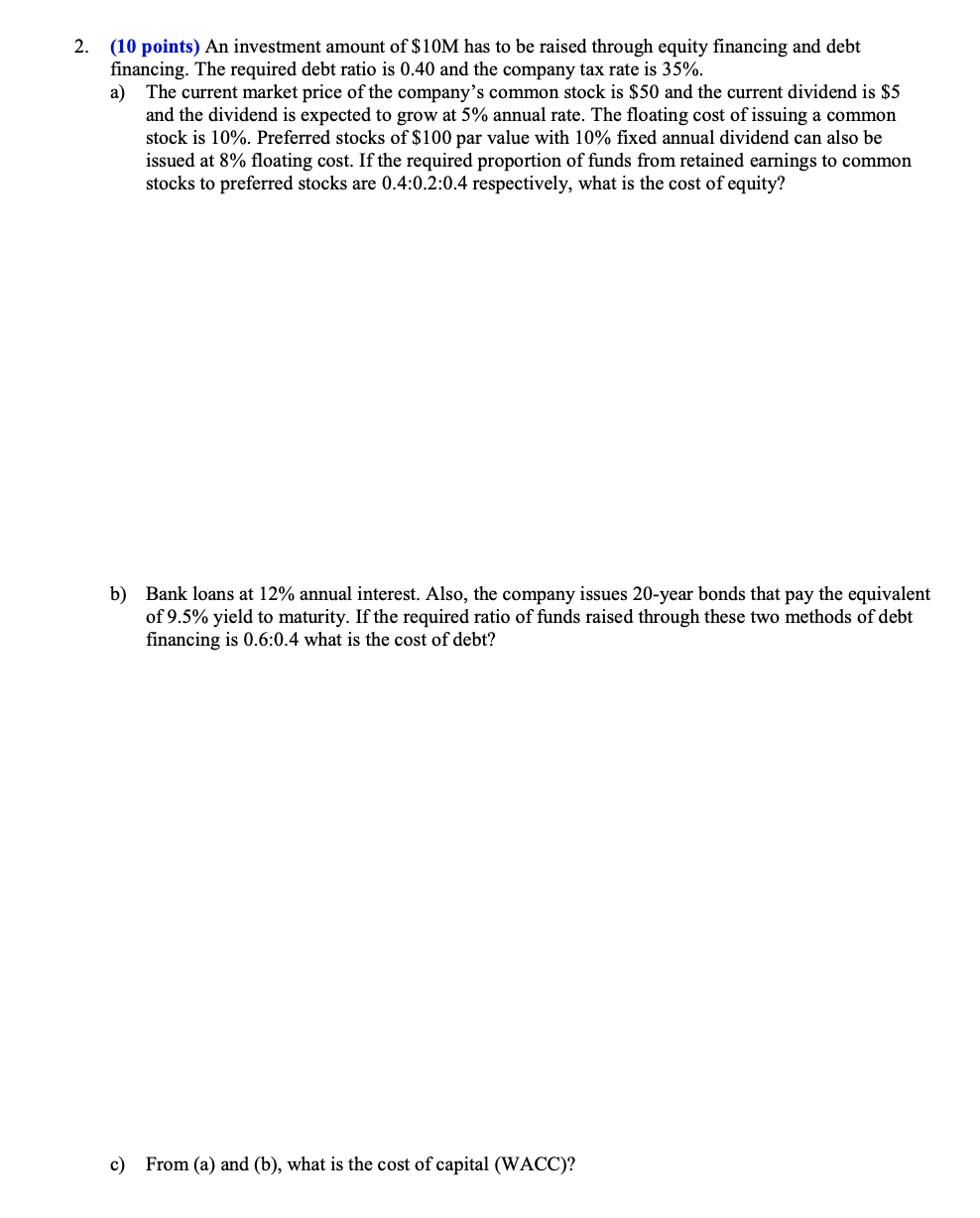Question

1. As the fraction of debt financing in a firm increases ................... a. the cost of...

1. As the fraction of debt financing in a firm increases ...................

a. the cost of debt increases

b. the cost of equity increases

c. the cost of equity decreases

d. the cost of preferred stock decreases.

Correct answer: b. the cost of equity increases

As the fraction of debt financing in a firm increases the cost of equity increases because increase of debt in capital structure of firm increases the financial risk of firm thus equity holders require a higher rate of return.

Hope it will help, please do comment if you need any further explanation. Your feedback would be highly appreciated.

Earn Coins

Coins can be redeemed for fabulous gifts.

Similar Homework Help Questions
• 1. Which of the following statements about lease financing is incorrect: Lease financing increases the risk...

1. Which of the following statements about lease financing is incorrect: Lease financing increases the risk for the firm. Lease financing can be considered a form of debt financing. Lease financing is subordinated to equity. Lease financing is typically considered a capital structure component. 2. Hybrid financing lowers the WACC for the firm. will result in dilution for the shareholders. is more risky then equity. Both A. and B. are correct. 3. What do lease financing and hybrid financing in...

• The cost of debt is usually lower than the cost of equity. The reason firms don't...The cost of debt is usually lower than the cost of equity. The reason firms don't use only debt financing is: Multiple Choice as the use of debt increases, the cost of debt decreases until no debt financing is available as the use of debes, so does the cost of equity as the use of debt increases, so does the tax rebate that the firm receives as the use of debt increases, government regulation increases

• Equity Lightning Corp. wishes to explore the effect on its cost of capital of the rate...

Equity Lightning Corp. wishes to explore the effect on its cost of capital of the rate at which the company pays taxes. The firm wishes to maintain a capital structure of 30% debt, 10% preferred stock, and 60% common stock. The cost of financing using retained earnings is 14%, the cost of preferred stock financing is 9%, and the before-tax cost of debt financing is 11%. Calculate the weighted average cost of capital (WACC) given the following tax rate assumptions:...

• Homework Problems: Fill in the table using the following information. Assets required for operation: \$10,400 Firm A uses only equity financing Firm B uses 40% debt with an 8% interest rate and 60% equity Firm C uses 50% debt with a 10% interest rate and 5Fill in the table using the following information.Assets required for operation: \$10,400Firm A uses only equity financingFirm B uses 40% debt with an 8% interest rate and 60% equityFirm C uses 50% debt with a 10% interest rate and 50% equityFirm D uses 50% preferred stock financing with a dividend rate of 10% and 50% equity financingEarnings before interest and taxes: \$1,040If your answer is zero, enter "0". Round your answers for monetary values to the nearest cent. Round your...

• The is the interest rate that a firm pays on any new debt financing. Wat after-tax...The is the interest rate that a firm pays on any new debt financing. Wat after-tax cost of debt VPC) can borrow funds at an interest rate of 10.20% for a period of five years. Its marginal federal-plus-state tax rate is 25% before-tax cost of debt Pebt is __ (rounded to two decimal places). At the present time, Water and Power Company (WPC) has 15-year noncallable bonds with a face value of \$1,000 that are outstanding. These bonds have a...

• An investment amount of \$10M has to be raised through equity financing and debt financing. The...An investment amount of \$10M has to be raised through equity financing and debt financing. The required debt ratio is 0.40 and the company tax rate is 35%. a) The current market price of the company’s common stock is \$50 and the current dividend is \$5 and the dividend is expected to grow at 5% annual rate. The floating cost of issuing a common stock is 10%. Preferred stocks of \$100 par value with 10% fixed annual dividend can also...

• Which of the following is not a cost to the firm of increasing debt financing? Group...

Which of the following is not a cost to the firm of increasing debt financing? Group of answer choices Investors will demand a higher interest rate on debt. The risk to common stockholders will increase. Stockholders will demand a higher return. The cost of common equity will decrease.

• 1-if a firm has 1500000 debt limit before AT kd will change and if taxes are...

1-if a firm has 1500000 debt limit before AT kd will change and if taxes are 40% and total equity in capital structure is 40% and the rest is debt calculate the debt break point in the MCC schedule interest is tax deductible a) \$1000000 b) \$7500000 c)\$2500000 d)\$3750000 2) the cost of internal common equity is equal to a) the cost of retaind earnings b) the cost of new common stock c) the cost of preferred stock d) the...

• Debt Financing and Equity Financing

Sand Key Development Company has a capital structure consisting of \$20 million of 10% debt and \$30 million of common equity. The firm has 500,000 shares of commonstock outstanding. Sand Key is planning a major expansion and will need to raise \$15 million. The firm must decide whether to finance the expansion with debt orequity. If equity financing is selected, common stock will be sold at \$75 per share. If debt financing is chosen, 9% coupon bonds will be sold....

• The Color Box uses a combination of common stock, preferred stock, and debt financing. The company...

The Color Box uses a combination of common stock, preferred stock, and debt financing. The company wants preferred stock to represent 7 percent of the total financing. It also wants to structure the firm in a manner that will produce a weighted average cost of capital of 9.5 percent. The aftertax cost of debt is 4.8 percent, the cost of preferred is 8.9 percent, and the cost of common stock is 14.7 percent. What percentage of the firm's capital funding...